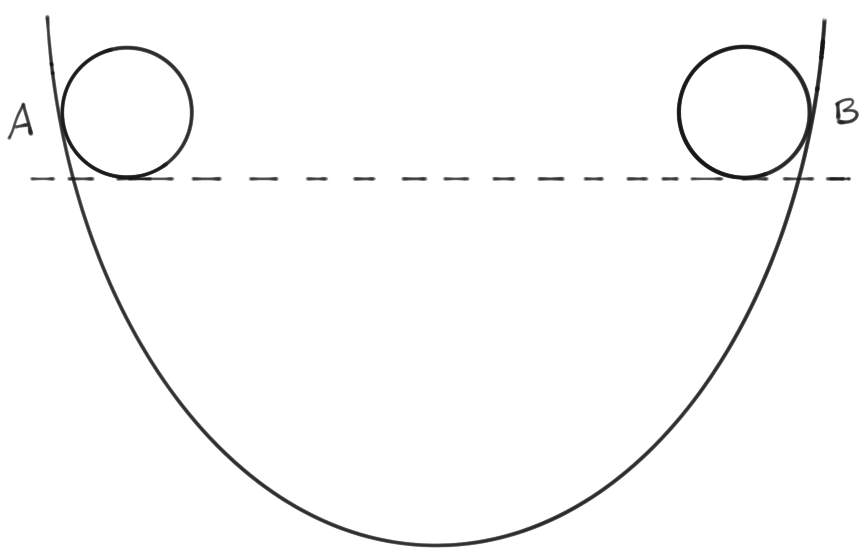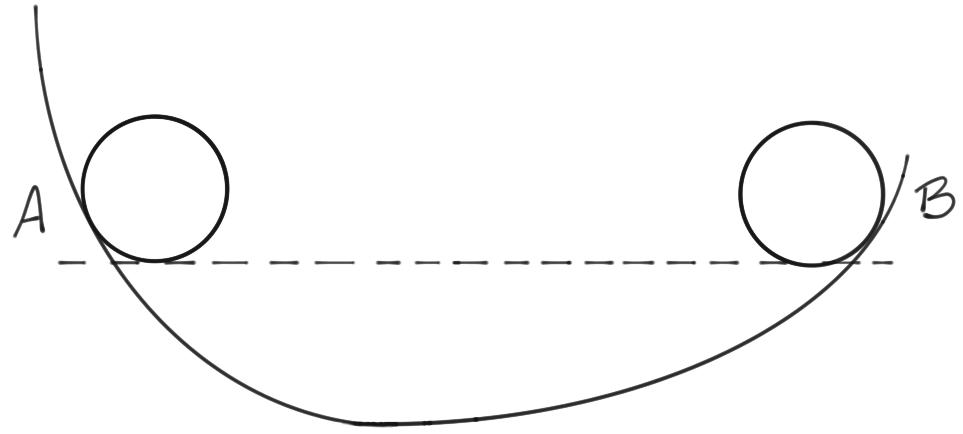Newton's First Law

# Newton’s first law of motion

To many, Newton’s three famous laws seem too trivial to be iconic. Yet, these form the basis of physics applicable in everyday life—often termed classical physics1. The key factor one needs to understand here is why these laws are important from a historic perspective: they signify one of the earliest applications of mathematics to studying the physical world and they stand as one of the earliest examples of a scientific approach taken to answer a question.

These three laws speak about the world around us; specifically they describe how things move—the dynamics of a body. In this article, we will be taking a look at Newton’s first law.

# Statement of the law

Newton’s first law describes inertial frames of reference by asserting their existence. This is why it is also known as the law of inertia. However, this definition is not clear outwardly from the statement of the law:

An object at rest stays at rest, and an object in motion remains in motion with a uniform velocity, unless acted upon by an unbalanced force.

We can split the law into three clauses:

1. An object at rest remains at rest
2. An object in motion remains in motion at a constant speed, in a constant direction
3. The states of rest or motion are disrupted only by an unbalanced force

An unbalanced force is one with no neutralising effect. For instance, a force of $x$ units northwards needs a force of $x$ units southwards—or perhaps two forces of $(x/\sqrt{2})$ units each, one southeastwards and one southwestwards, and so on—to balance it out. Should such balancing forces not exist, we call the existing force an unbalanced force.

# Outcomes of the law

## Inertia

All this tells us about the habit of things in our universe is that they adamantly remain in their present state. More scientifically, this tells us how an object prefers to retain its state of rest or motion so long as some unbalanced force does not disturb it. This preference, or resistance of an object to any change in its state of rest or motion is termed inertia.

This was a powerful idea because in Newton’s time it was largely believed that objects preferred to come to rest. Nobody bothered about a force like friction that they could not ‘see’, so to speak2.

## Friction

However, the idea of friction must rightly be attributed to Galileo who believed that such a force existed and worked towards bringing moving things to rest. It is interesting how Galileo arrived at his idea empirically, i.e. using experiments rather than a logical thought process.Galileo's experimentFig. 1: All things considered equal a ball released at A goes up to, and no more or less that, B at the same height above ground as A.

From fig. 1 it is clear that an object released at position A on some container would reach position B at the same height as A — under ideal circumstances. Galileo observed as much.Fig. 2: All things considered equal a ball released at A goes up to, and no more or less that, B at the same height above ground as A.

As in fig. 2, Galileo modified his experiment and found that this height would be reached regardless of the angle of inclination, i.e. no matter how wide or narrow the container got or how asymmetric his set-up was.Fig. 3: Logic dictates then that in this case the ball must keep traveling—perhaps infinitely—until it reaches the same height as A, yet we know from experience that the ball will eventually come to a halt. Why?

Finally, in fig. 3, the genius of Galileo shows: he reasoned that if the angle was a full 180 degrees, meaning that if the slope never brought the object up to its initial position, the object should keep moving, never being able to attain its desired height. Unsurprisingly he found the object does stop. So, he reasoned, there must be some unseen force in whose nature it is to stop moving objects. This is the force we now call friction.

However, Galileo’s idea was rudimentary. We know today that the reason you can walk, putting one step before the other and not slipping every time, is because friction prevents your feet from slipping. In essence friction helps you walk; what it prevents is any sort of motion (the slippage) between the ground and your feet.

So, in the case of a ball that is rolling on the ground friction brings it to rest and, in the case of your feet about to slip, friction keeps it at rest. It can be tempting to conclude, not unlike Galileo, that friction prevents motion but the idea goes a little deeper: what friction prevents is relative motion; it stops relative motion between the ball and the ground and prevents it altogether between your feet and the ground. This is the real nature of friction:

Friction is a force that attempts to prevent any relative motion between two bodies.

With this we come to the final outcome of Newton’s powerful first law.

# Mass

Think of the individuality of an object: do some objects have more inertia than others? In other words, do some have a greater preference to remain at rest or motion? The answer is simply that they do, and this degree of preference that an object has to remain at its present state is given the term mass. Mass is also defined, when more convenient, as the amount of matter contained in a body; but it is more scientifically described as the measure of the tendency of an object to oppose a change in its state of rest or motion.

Suppose a force $\mathbf{F}$ causes a body to accelerate at the rate $\mathbf{a}$. Newton’s first law tells us that the greater the force is the greater the acceleration is and vice versa. This is why when $\mathbf{F} = 0$ for a body $\mathbf{a} = 0$ too. Subsequently,

\begin{align*} \mathbf{F} &\propto \mathbf{a} \nonumber \\[.5em] \implies \mathbf{F} &= m \mathbf{a} \tag{1} \label{eq:F=ma} \end{align*}

where $m$ is a proportionality constant known as the inertial mass of the object in question.

What eq. $\eqref{eq:F=ma}$ tells us is that the more massive an object the greater is the force required to reduce its relative motion, when the acceleration remains constant. Alternatively, if two bodies are being accelerated at the same rate more force would be needed to bring the more massive one to relative rest. In other words mass is the measure of inertia of an object.

# Inertial frames of reference

Now there is a catch to this law. Consider a bowling ball rolling along a polished wooden floor on a ship. The ball keeps rolling until some force stops it. This force could be friction with the floor, collision with a wall etc. Assuming the floor is perfectly polished (frictionless) we realise that the ball keeps moving at a uniform velocity.

Suppose the ship (and hence the entire room) was accelerating away from some point, then the bowling ball would, with respect to that point, no longer have a constant velocity, not because of any force—in fact there is no force involved at all—but because of a change in speed due to acceleration, thereby rendering Newton’s law invalid.

It is important to note, therefore, that the fact that this law can be experimentally verified tells us that frames of reference exist (such as our bowling room in the last example) wherein this law remains true. Such frames are called inertial frames of reference and hence this law is sometimes also called the law of inertia. Frames where this law does not apply are called non-inertial reference frames.

The law of inertia has a huge number of experimental verifications in daily life. Consider the way you fall forwards or backwards when a car brakes to a halt or accelerates at a traffic light. That is due to inertia, when your body would rather remain moving forwards than slow to a halt, or vice versa.

It is for the same reason that you would have a nasty fall if you let go of a fast-moving swing in the park. Although the fall and the path you take before your face hits the ground are governed by other forces, what makes you leave the swing and fly into the air is inertia.

Although not direct, the ideas that this law introduces go on to become pivotal in framing physics. From rotational dynamics to cosmology, the concepts of mass and inertia play a key role almost everywhere.

1. This is physics that works at everyday speeds for macroscopic objects. [return]
2. It is no surprise that Newton hit upon inertia which was essentially an ‘invisible’ force. In fact he more famously talked about another invisible force, gravity, further in his career. [return]
By V.H. Belvadi